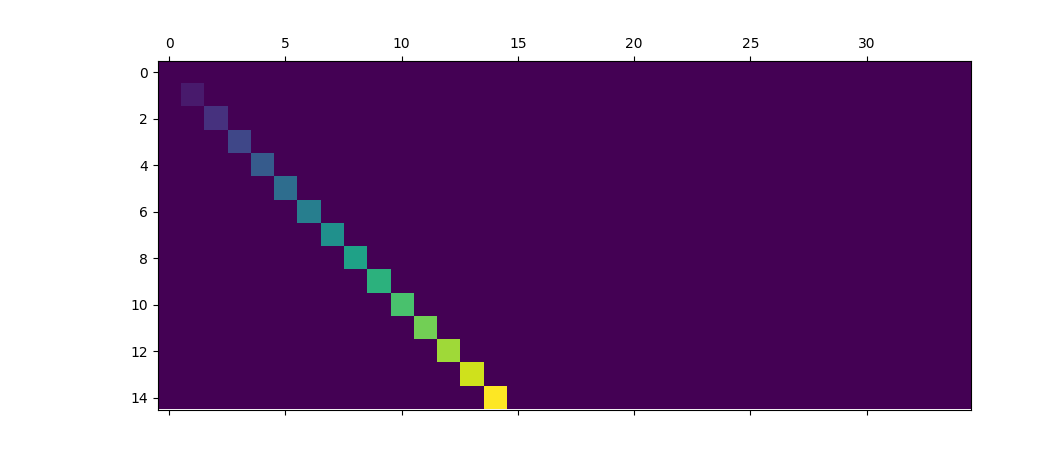Travis-CI:# pylab_examples example code: matshow.py¶```"""Simple matshow() example."""
import matplotlib.pyplot as plt
import numpy as np

def samplemat(dims):
"""Make a matrix with all zeros and increasing elements on the diagonal"""
aa = np.zeros(dims)
for i in range(min(dims)):
aa[i, i] = i
return aa

# Display matrix
plt.matshow(samplemat((15, 35)))

plt.show()
```

Keywords: python, matplotlib, pylab, example, codex (see Search examples)